## A handy app to convert between US, imperial and metric units: Length Converter, Area Converter, Time converter, Temperature converter, Weight Converter, Volume Converter, Speed Converter, Power Converter, Pressure Converter, Energy Converter, Fabric Converter, Fuel consumption converter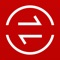# Unit Converter 12in1

by Intemodino Group s.r.o.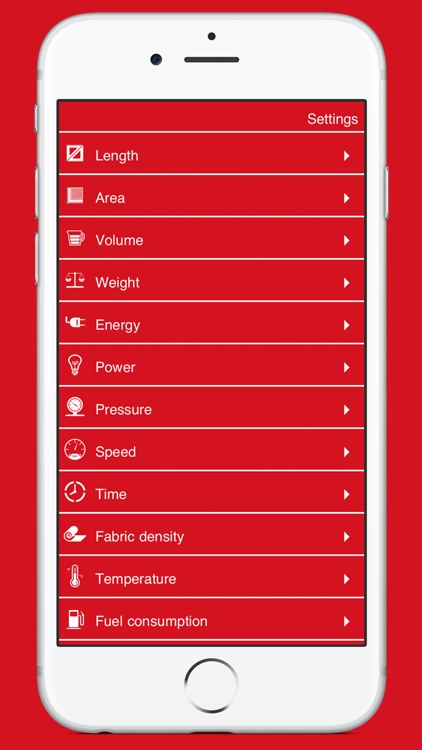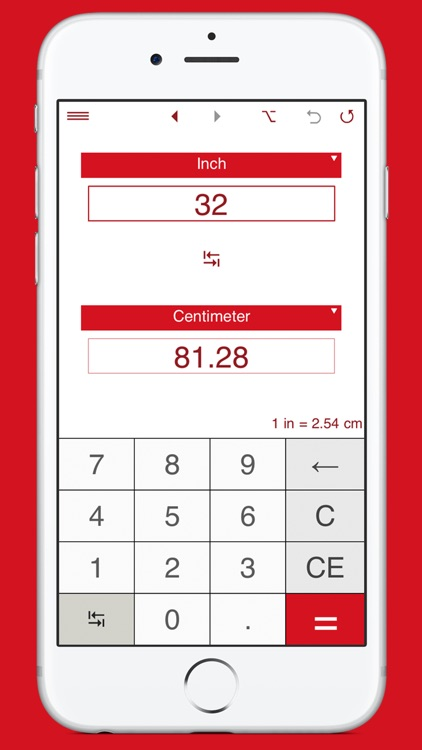A handy app to convert between US, imperial and metric units: Length Converter, Area Converter, Time converter, Temperature converter, Weight Converter, Volume Converter, Speed Converter, Power Converter, Pressure Converter, Energy Converter, Fabric Converter, Fuel consumption converter.### App Details

Version
2.1.0
Rating
(7)
Size
26Mb
Genre
Productivity Utilities
Last updated
February 10, 2020
Release date
December 9, 2015

### App Screenshots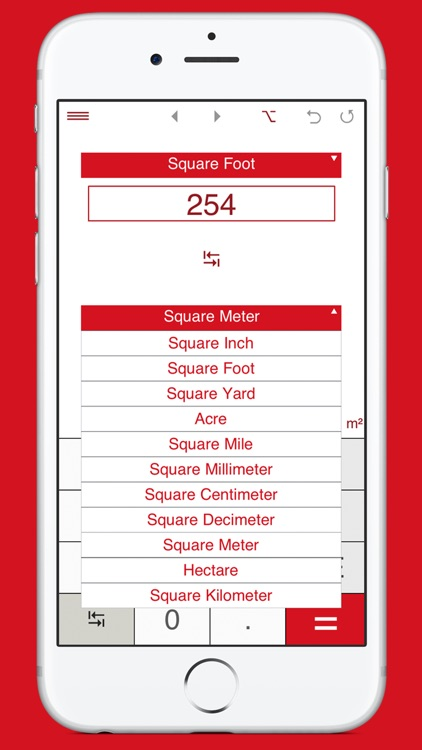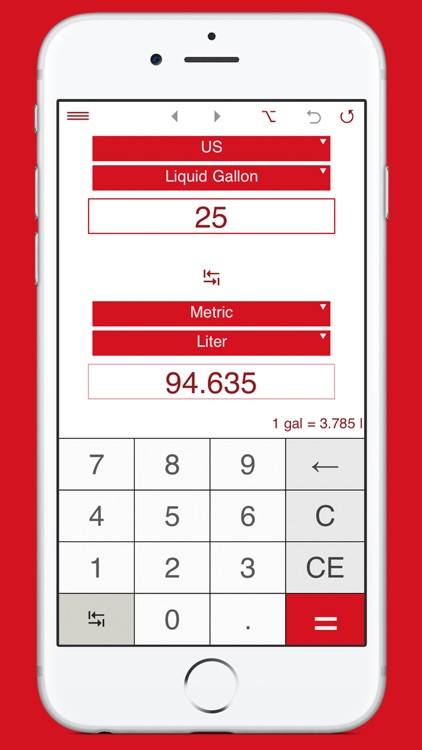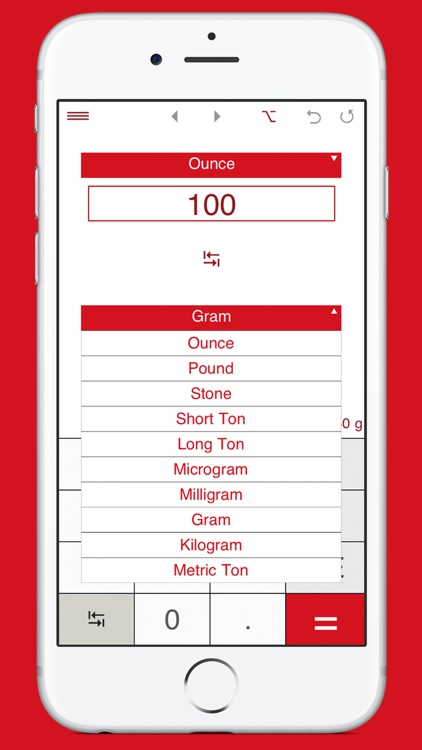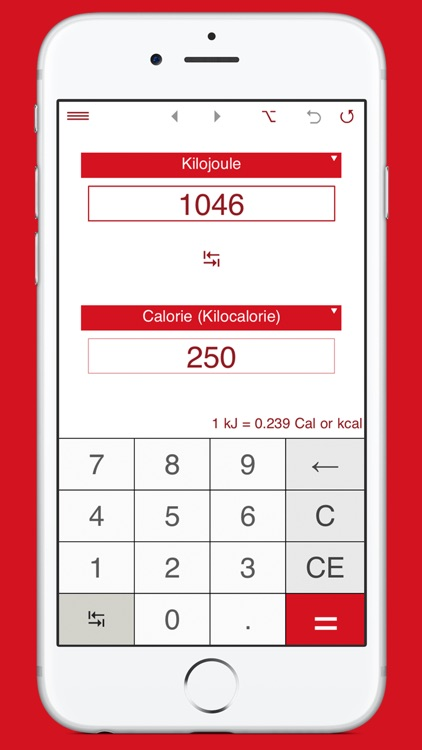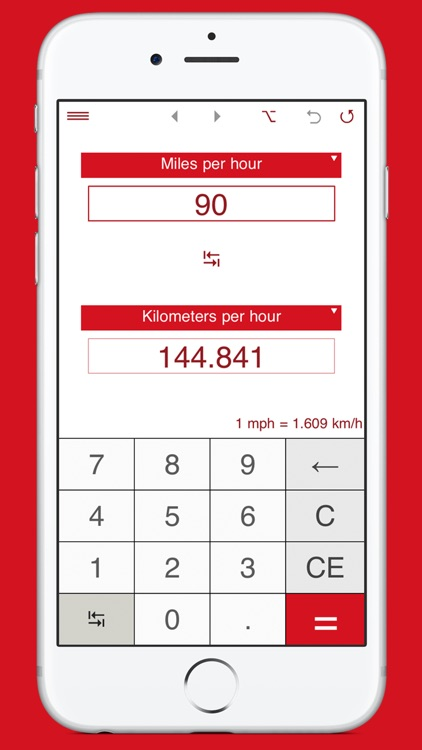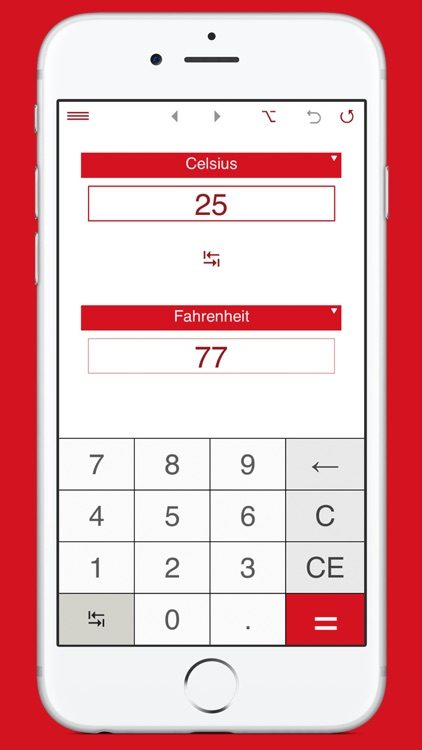### App Store Description

A handy app to convert between US, imperial and metric units: Length Converter, Area Converter, Time converter, Temperature converter, Weight Converter, Volume Converter, Speed Converter, Power Converter, Pressure Converter, Energy Converter, Fabric Converter, Fuel consumption converter.

Length Converter
Supported length units:
• Inch
• Foot
• Yard
• Mile
• Mile (nautical)
• Kilometer
• Meter
• Decimeter
• Centimeter
• Millimeter

Area Converter
Supported area units:
• Square Inch
• Square Foot
• Square Yard
• Acre
• Square Mile
• Square Millimeter
• Square Centimeter
• Square Decimeter
• Square Meter
• Hectare
• Square Kilometer

Time converter
Supported time units:
• Nanosecond
• Microsecond
• Millisecond
• Second
• Minute
• Hour
• Day
• Week
• Month
• Year
• Century

Temperature converter
Supported temperature units:
• Celsius °C
• Fahrenheit °F
• Kelvin K

Weight Converter
Supported mass units:
• Ounce (US)
• Pound (US)
• Stone
• Short Ton (US)
• Long Ton (UK)
• Microgram
• Milligram
• Gram
• Kilogram
• Metric Ton

Volume Converter
Supported volume units:
US volume units:
• Barrel (petroleum)
• Barrel (fluid)
• Barrel (beer)
• US liquid gallon
• US liquid quart
• US liquid pint
• US cup
• US gill
• US fluid ounce
• US tablespoon
• US teaspoon
• US fluid dram
• Cubic foot
• Cubic inch

Imperial volume units:
• Barrel (petroleum)
• Barrel
• Imperial gallon
• Imperial quart
• Imperial pint
• Imperial cup
• Imperial gill
• Imperial fluid ounce
• Imperial tablespoon
• Imperial teaspoon
• Imperial drachm
• Cubic foot
• Cubic inch

Metric volume units:
• Сubic meter
• Сubic decimeter
• Сubic centimeter
• Сubic millimeter
• Liter
• Deciliter
• Centiliter
• Milliliter

Speed Converter
Supported speed units:
• Miles per hour (mi/h or mph)
• Miles per minute (mi/min)
• Miles per second (mi/s)
• Feet per minute (fpm or ft/min)
• Feet per second (fps or ft/s)
• Inches per second (ips)
• Knots or nautical miles per hour (kn or kt)
• Kilometers per hour (km/h or kph)
• Kilometers per minure (km/min)
• Meters per minute (m/min)
• Meters per second (m/s)

Power Converter
Supported power units:
• Watt
• Kilowatt
• Horsepower (electric) hp(E)
• Horsepower (metric) hp(M)
• Foot-Pounds per hour (ft-lbf/h)
• Foot-Pounds per minute (ft-lbf/min)
• Foot-Pounds per second (ft-lbf/s)
• Btu per minute (Btu/min)
• Erg per second (Erg/s)

Pressure Converter
Supported pressure units:
• Atmosphere
• Millibar
• Bar
• Pascal
• Hectopascal
• Kilopascal
• Pounds per square inch (PSI)
• Pounds per square foot (PSF)
• Kilograms per square meter kgf/m²
• Inches of mercury (inHg)
• Millimeters of mercury (mmHg)
• Torr

Energy Converter
Supported energy units:
• Joule
• Kilojoule
• Calorie
• Kilocalorie
• Watt hour
• Kilowatt hour
• Electronvolt
• British thermal unit (BTU)
• Therm (US)
• Foot pound

Fabric Converter
You can easily convert:
- Grams per Square Meter (g/m2) to Ounces per Square Yard (oz/yd2).
- Ounces per Square Yard (oz/yd2) to Grams per Square Meter (g/m2).

Fuel consumption converter
Supported units:
Kilometers per liter (km/L)
Liters per 100 kilometers (L/100 km)
Miles per gallon (US) (US MPG)
Miles per gallon (UK) (UK MPG)

"Flip" button to easily switch between the "from" and "to" units.

Supports fractions.

Results are shown in the decimal and fractional forms.

App's Settings:
- Rounding to specified number of decimal places: for decimal numbers, you can select the number of decimal places you want to display. By default, the app displays 2 decimal places.
To round results to the nearest whole number, select 0 decimal places.

- For fractions, you can select rounding to the nearest 1/2, 1/4, 1/8, 1/12, 1/16, 1/32, 1/64, 1/128, 1/256. By default, the app rounds fractions to the nearest 1/32.
If you do not need to round fractions, just select "-".

Sends results and history via email.

Conversion history.

Disclaimer:
AppAdvice does not own this application and only provides images and links contained in the iTunes Search API, to help our users find the best apps to download. If you are the developer of this app and would like your information removed, please send a request to [email protected] and your information will be removed.